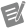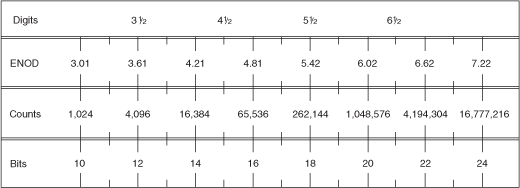# Resolution

NI Digital Multimeters Help (NI-DMM 18.1)

Edition Date: March 2018

Part Number: 370384V-01

»View Product InfoDownload Help (Windows Only)

For a noise-free DMM, resolution is the smallest change in an input signal that produces, on average, a change in the output signal. Resolution can be expressed in terms of bits, digits, or absolute units, which are related to each other.

### Bits

Resolution is often expressed in bits, which specifically refers to the performance of the analog-to-digital converter (ADC). Theoretically, a 12-bit ADC can convert an analog input signal into 212 (4,096) distinct values. 4,096 is the number of least significant bits (LSB). LSB can be translated into digits of resolution:

Digits of resolution = log10 (Number of LSB)

Using the previous equation, a DMM with a 12-bit ADC has a resolution of:

Log10 (4,096) = 3.61 digitsNote  If a 12-bit ADC is used to digitize signals in a DMM, it might be insufficient to call this DMM a 3½–digit DMM, as noise must also be considered. Noise may reduce the number of LSBs and, therefore, the number of digits.

### DMM Absolute Units and Digits of Resolution

For traditional DMMs, 5½ digits refers to the number of digits displayed on the readout of a DMM. A traditional 5½–digit DMM has five full digits that only display values from 0 to 9 and one half digit that only displays 0 or 1. This DMM can show positive or negative values from 0 to 199,999.

For more sophisticated digital instruments, and particularly virtual instruments, digits of resolution does not directly apply to the digits displayed by the readout.

#### Absolute Units

Counts for a DMM is analogous to LSBs for an ADC. A count represents a value that a signal can be digitized to and is equivalent to a step in a quantizer. The weight of a count, or the step size, is the absolute unit of resolution.

Absolute unit of resolution = total span/counts

#### Digits

Digits can be defined as:

Digits of resolution = log10 (total span/absolute unit of resolution)

For example, a noise–free DMM set to the 10 V range (20 V total span) with 200,000 available counts has an absolute unit of resolution of:

Absolute unit of resolution = 20.0 V/200,000 = 100 µV

The readout of this noise–free DMM would display six digits. A change of the last digit would indicate a change of 100 µV of the input signal.

An 18-bit ADC provides the minimum number of LSB. You can now calculate the digits of resolution:

(217 = 131,072, 218 = 262,144)

Digits of resolution = log10 (20.0 V/100 x 10-6 V)

Digits of resolution = 5.3

This noise–free DMM can be called a 5½ digit DMM.

The quantization process introduces into any converted signal an irremovable error, called the quantization noise. For input signals through a uniform quantizer (without overload distortion), the rms value of the quantization noise in a noise–free DMM can be expressed as:

rms of quantization noise = absolute units of resolution/√12

In reality, a noise-free DMM does not exist and you need to account for the noise level when calculating absolute units of resolution. You can define the effective absolute units of resolution of a noisy DMM as the step size of a noise–free DMM with a quantization noise equal to the total noise of the noisy DMM.

Effective absolute units of resolution = √12 * rms noise

You can define the Effective Number of Digits (ENOD) of this noisy DMM as:

ENOD = log10(total span/Effective absolute units of resolution)

#### Example 1

If a DMM set on the 10 V range (20 V total span) shows readings with an rms noise level of 70 µV, its effective absolute units of resolution and the ENOD is:

Absolute units of resolution = √12 * 70 µV = 242.49 µV

ENOD = log10 (20.0 V/242.49*10-6 V) = 4.92 digits

This DMM can be called a 5 digit DMM.

The minimum number of counts needed for this DMM would be 20 V/242.49*10-6 V = 82,478. The minimum number of bits needed would be 17 (216 = 65,536, 217 = 131,072).

#### Example 2

If the same DMM demonstrates an rms noise level of 20 µV:

Absolute units of resolution = √12 * 20 µV = 69.28 µV

ENOD = log10 (20 V/69.28*10-6 V) = 5.46 digits

This DMM can be considered a "5½" digit DMM.

The minimum number of counts needed for this DMM would be 20 V/69.28*10-6 V = 288,675. The minimum number of bits needed would be 19 (218 = 262,144, 219 = 524,288).

The following table relates bits, counts, and ENOD to conventional digits of resolution for DMMs. As evidenced by the table, bits, counts, and ENOD are deterministically related. A direct mathematical relationship between ENOD and digits does not exist because digits are used only as an approximation.